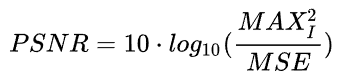# How to calculate PSNR after normalization

We know that PSNR as below:whereandSo if I normalize the image before calculating PSNR, I won’t know. In this case, how to calculate PSNR?